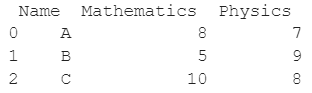# How To Get A List From Pandas DataFrame Column Headers?

A DataFrame is the primary data structure of the Pandas library and is commonly used for storing and working with tabular data. A common operation that could be performed on such data is to get a list of column headers in order to extract information from it.

To start working with Pandas, we first need to import it in the Python code:

### Python 3 Code :

``import pandas as pd``

## Running Example

Let us understand this operation with the help of an example. Consider the following DataFrame containing 3 students with names A, B, and C and their corresponding marks (out of 10) for two subjects, Mathematics and Physics.Code snippet for generating the above DataFrame :

### Python 3 Code :

``````import pandas as pd
# Dictionary for our data
data = {'Name' : ['A', 'B', 'C'], 'Mathematics' : [8, 5, 10], 'Physics' : [7, 9, 8]}
# DataFrame for the dictionary
df = pd.DataFrame(data)
# Printing the DataFrame
print(df)``````

Here, data is a dictionary we created to initialize the DataFrame. For this, we use the DataFrame() function of the Pandas library which takes the dictionary as an argument and returns the required DataFrame.

Now let us say that for some reason, we want to get a list from the column headers of this DataFrame df, the resulting output will look as follows :

['Name', 'Mathematics', 'Physics']

Let us look at different ways of performing this operation on a given DataFrame :

## Using the list() function to get list of DataFrame header

In this method, we use the list() function to get a list from the column headers of a given Pandas DataFrame.

On taking the DataFrame df as a parameter, the list() function returns a list of the column headers which we assign to the variable col_headers.

Let us take a look at the code and corresponding output for this method. The list() function is basically used for type-casting here.

### Python 3 Code :

``````import pandas as pd
# Dictionary for our data
data = {'Name' : ['A', 'B', 'C'], 'Mathematics' : [8, 5, 10], 'Physics' : [7, 9, 8]}
# DataFrame for the dictionary
df = pd.DataFrame(data)
# Getting the list of column headers
# Printing the list

### Output :

['Name', 'Mathematics', 'Physics']

## Using the list() function with DataFrame.columns property input

In this method, we use the list() function to get a list from the column headers of a given Pandas DataFrame. df.columns return the columns of the DataFrame.

On taking the df.columns property as a parameter, the list() function returns a list of the column headers which we assign to the variable col_headers.

Let us take a look at the code and corresponding output for this method. The list() function is basically used for type-casting here.

### Python 3 Code :

``````import pandas as pd
# Dictionary for our data
data = {'Name' : ['A', 'B', 'C'], 'Mathematics' : [8, 5, 10], 'Physics' : [7, 9, 8]}
# DataFrame for the dictionary
df = pd.DataFrame(data)
# Getting the list of column headers
# Printing the list

### Output :

['Name', 'Mathematics', 'Physics']

## Using the tolist() function with DataFrame.columns property

In this method, we use the .tolist() function to get a list from the column headers of a given Pandas DataFrame. The df.columns return the columns of the DataFrame. On applying the .tolist() function to this returned object, a list containing the column headers of the DataFrame is returned.

Let us take a look at the code and corresponding output for this method. The tolist() function is basically used for type-casting here.

### Python 3 Code :

``````import pandas as pd
# Dictionary for our data
data = {'Name' : ['A', 'B', 'C'], 'Mathematics' : [8, 5, 10], 'Physics' : [7, 9, 8]}
# DataFrame for the dictionary
df = pd.DataFrame(data)
# Getting the list of column headers
# Printing the list

### Output :

['Name', 'Mathematics', 'Physics']

## Using the tolist() function with DataFrame.columns.values property

In this method, we use the tolist() function to get a list from the column headers of a given Pandas DataFrame. The df.columns.values property returns the column values of the DataFrame. On applying the .tolist() function to this returned object, a list containing the column headers of the DataFrame is returned.

Let us take a look at the code and corresponding output for this method. The .tolist() function is basically used for type-casting here.

### Python 3 Code :

``````import pandas as pd
# Dictionary for our data
data = {'Name' : ['A', 'B', 'C'], 'Mathematics' : [8, 5, 10], 'Physics' : [7, 9, 8]}
# DataFrame for the dictionary
df = pd.DataFrame(data)
# Getting the list of column headers